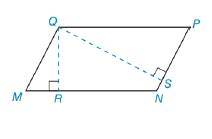Chapter 8.1, Problem 49EElementary Geometry For College St...

7th Edition
Alexander + 2 others
ISBN: 9781337614085

Solutions

Chapter
SectionElementary Geometry For College St...

7th Edition
Alexander + 2 others
ISBN: 9781337614085
Textbook Problem

In Exercises 47 to 50, use the fact that the area of the polygon is unique.In ▱ M N P Q ,   Q P = 12 and Q M = 9. The length of altitude Q R ¯ (to side M N ¯ ) is 6. Find the length of a altitude Q S ¯ from Q to P N ¯ .To determine

To find:

The length of the altitudes

Explanation

Converting theoretical statement into mathematical statement, identifying the geometric figure and the calculation is done by suitable geometric formulae.

Calculation:

Given,

Here, MN=b=12

QR=h=6

Area of the parallelogram A=bh unit2

=(12)(6)

=72 unit2

Find QS¯

For Q to PN¯

Choosing

Still sussing out bartleby?

Check out a sample textbook solution.

See a sample solution

The Solution to Your Study Problems

Bartleby provides explanations to thousands of textbook problems written by our experts, many with advanced degrees!

Get Started

Find the critical numbers of the function. F(x) = x4/5(x 4)2

Single Variable Calculus: Early Transcendentals, Volume I

Evaluate the integral. 70. 11+2exexdx

Single Variable Calculus: Early Transcendentals

Elimination of the parameter in x = 2t3/2, y = t2/3 gives: x4 = 16y9 16x4 = y4 x3 = 8y4 8x3 = y4

Study Guide for Stewart's Single Variable Calculus: Early Transcendentals, 8th